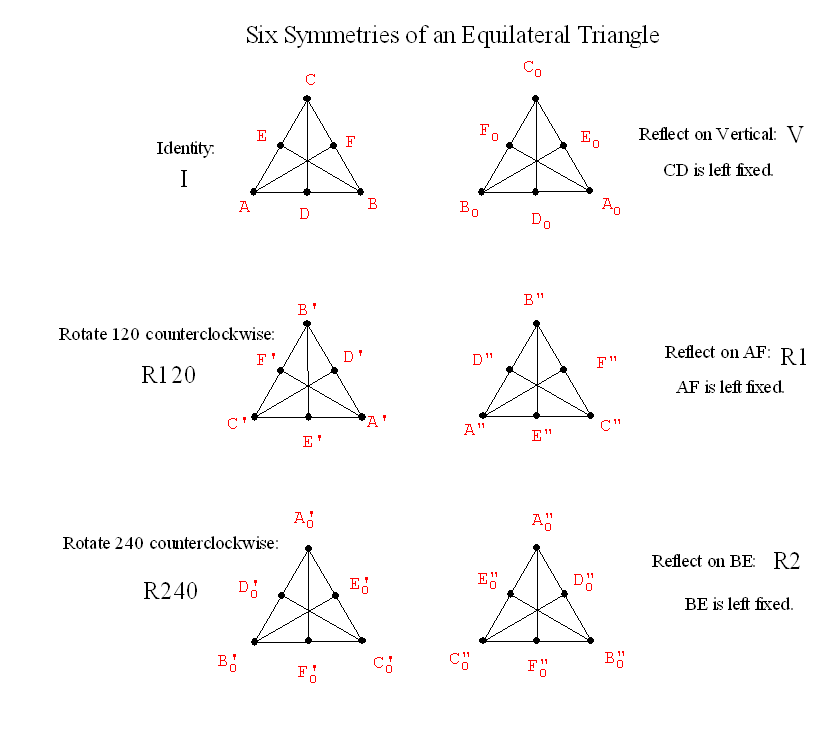# Tuesday  September 20

REVIEW: Recall our previous discussions for a polygon with n sides.

In general: the sum of the interior angles in a n sided polygon is
(n-2) *180 degrees.

A regular  polygon is a polygon where the sides are all of equal length and the angles are all congruent (or of equal measure).

In general: The individual angle for a regular polygon with n sides is (n-2)*180/n degrees.
This can be expressed in other ways using algebra:
(n-2)*180/n = [180 n - 360] / n = 180 - 360/n.

 name of polygon n degrees of the interior  measure of each angle 360 degrees divided  by # in Column 2 equilateral triangle 3 60 360 / 3 = 120 square 4 90 360/4= 90 regular pentagon 5 3*180/5= 108 360/5= 72 regular hexagon 6 4*180/6=120 360/6= 60 regular heptagon 7 5*180/7 360/7 regular octagon 8 6*180/8=135 360/8 = 45 regular dodecagon 12 10*180/12=1800/12=150 360/12=30

• Dissection of the plane--- Tilings of the plane.

• One polygonal Tile: Quadrilateral Activity.
• An on-line tool for making tilings of the plane
• Regular and semiregular Tilings of the plane.
• A tiling is a regular tiling if
(i) it has a single tile shape that is a regular polygon and
(ii) the vertices and edges of the tiles coincide (no overlapping edges)
• A tiling is a semi-regular tiling if
(ii) each tile shape is a regular polygon,
• (ii) the vertices and edges of the tiles coincide (no overlapping edges) and
• (iii) every vertex has the same polygon types arranged around it.
• Naming tilings (Math Forum)
• The numbers represent the number of sides in the poygons.
• The order indicates the order in which the poygons are arranged about a vertex.

• Recall Symmetries of an equilateral triangle:• Combining transformations to give new symmetries:
Example: Let V denote reflection across the line that is the vertical altitude of the equilateral triangle.

And consider a second symmetry, called R=R120, which will rotate the equilateral triangle counterclockwise about its center O by 120 degrees.

We first perform V to the figure and then perform R to the figure.
We will denote this V*R... meaning V followed by R.

The resulting transformation also leaves the equilateral  covering the same position in which it started, and....

V*R     =   R2.

What about other products?  This gives a  "product" for symmetries.
If S and R are any symmetries of a figure then S*R is also a symmetry of the figure.

A "multiplication" table for Symmetries:
 * Id R120 R240 V G=R1 H=R2 Id I R120 R240 R1 R240 I R2 V R2 R1 I R120 G=R1 I H=R2 I
Do Activity on product of symmetries.
This shows that R240*V = R1

This "multiplicative" structure  is called the Group of symmetries of the equilateral triangle.

Given any figure we can talk about the group of its symmetries.
Does a figure always have at least one symmetry? .....

Yes... The Identity symmetry.
Such a symmetry is called the trival symmetry.